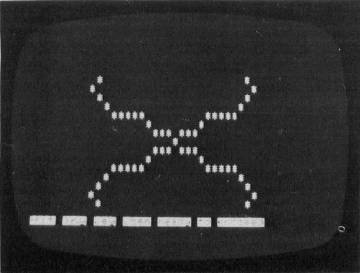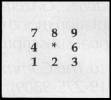`COMPUTE! ISSUE 34 / MARCH 1983 / PAGE 242`

PETterns

Bruce Shawyer

For PET/CBM's with 40-column screens, "PETterns" creates patterns, rotates and reflects them, and can be used to introduce mathematical concepts to students. Although the program can use the Commodore 2022 printer for hardcopy, it will also work with most other printers.

"PETterns" wiJl create patterns on the screen, rotate them, and reflect them. For any created pattern, the user can print it as it was created, print its rotation through 90°, 180°, or 270°, print its reflection in the positive diagonal line (its inverse), or print the rotations of the inverse. Certain combinations of these can be obtained directly in order to create pretty patterns.

The program is useful in introducing students to group theory, for the basis of the program is the group of symmetries of the reflections and rotations of the square (the dihedral group). This group has two generators, but they are, of course, not unique. For the first, I chose the rotation through 90° in the positive (counterclockwise) sense. For the other, I chose the reflection in the positive diagonal line (the line Y = X).

The other group operations are done within the program by calling the appropriate combinations of the generators. Thus they are slightly slower. I chose the second generator because it gives the inverse relation to the relation created, and so is useful in illustrating, for example, that the inverse of a function is not necessarily a function.A crystalline shape begins to form in "PETterns."

The program given here is designed for a 40-column screen PET and uses the alternate character set. For reasons of symmetry, patterns of 39-columns by 25-rows are allowed. This permits the right-hand column to be reserved for a set of prompts to the set of input codes.

Patterns start at the center of the screen. This point is the origin for the X- and Y-axes. A symbol, initially a "*", will appear at this point. To print the next point, use the numeric key-pad in the conventional way. "5" is the center. The other non-zero digits are used to enter the direction for the next point to be printed. For example, entering "9" results in the next point printed being in a north-east direction from the previous point.There are also five codes which allow combinations of the above to be printed. These codes are "a", "b", "c", "d" and "e".

Code "a" prints the original pattern together with reflections as if the X- and Y-axes were mirrors. That is, we have p, S, t and F.

Code "b" prints the inverse of the pattern together with reflections as if the X- and Y-axes were mirrors. That is, we have P, s, T and f.

Code "c" prints the original pattern together with its rotations. That is, we have p, s, t and f.

Code "d" prints the inverse of the pattern together with its rotations. That is, we have P, S, T and F.

Code "e" prints everything, that is, all eight possible positions.

There are two codes not appearing in the prompt column. The first is "h". This stands for "cHange the symbol". When you hit "h", the right-hand column is replaced by a list of symbols. Each has a number beneath it. By hitting the number that appears below a symbol, you cause that symbol to be the next printed in the patterns. This new symbol will continue until another symbol is selected. Note that "1" is below the blank symbol.

The second code is "v". This stands for "change Vector length". When you hit "v", the right-hand column is replaced by the words "CHANGE VECTOR LENGTH"; beneath them is the length of the present vector. When you hit a non-zero number, a new vector length is entered. When a new point is next entered, it will be repeated the number of times of the length of the vector.

Here is a list of other codes appearing in the prompt column, with an explanation of their effects:

 CODE EFFECT q QUIT - terminates the program. r RUN - restarts the program for a new pattern. n NOTHING - clears the screen. o OUTPUT - prints the pattern on the printer. This program is written for a CBM 2022 printer. P PRINT - prints your pattern on the screen. s SECOND - rotates the pattern through 90°. t THIRD - rotates through 180°. f FOURTH - rotates through 270°. P (Shift)p - reflects the pattern in the positive diagonal. S (Shift)s - reflects, then rotates 90°. T (Shift)t - reflects, then rotates 180°. F (Shift)f - reflects, then rotates 270°.